Your research can change the world
More on impact ›

# Frontiers in Nanotechnology## ORIGINAL RESEARCH article

Front. Nanotechnol., 07 April 2021 | https://doi.org/10.3389/fnano.2021.638442

# Strong Coupling, Hyperbolic Metamaterials and Optical Tamm States in Layered Dielectric-Plasmonic Media

• Institute of Experimental Physics, Ulm University, Ulm, Germany

Thin films of noble metals with thickness smaller than the wavelength of light constitute one of the most investigated structures in plasmonics. The fact that surface plasmon modes can be excited in these films by different ways and the simplicity of fabrication offer ideal conditions for applications in nanophotonics. The generation of optical modes in coupled Fabry-Pérot planar cavities and their migration to hyperbolic metamaterials is investigated. Coupled Fabry-Pérot cavities behave as simple coupled resonators. When the intra-cavity media have different refractive indices in two or more coupled cavities resonance anti-crossings arise. The application of this kind of strong coupling in sensing is foreseen. Beyond the cavity modes excited by propagating waves, also long range plasmonic guided modes can be excited using emitters or evanescent waves. A periodic structure made by multiple plasmonic films and dielectrica supports bulk plasmons, of large propagation constant and increasing field amplitude. The optical response of these structures approaches that of the hyperbolic metamaterial predicted by the effective medium theory. Light can propagate with full transmission in a structure made of a photonic crystal based on quarter wavelength layers and a second photonic crystal with an overlapping forbidden band, but presenting a non-trivial topological phase achieved by band inversion. This is due to excitation of optical Tamm states at the boundary between both crystals. The extension to multiple optical Tamm states using dielectric and plasmonic materials and the symmetries of the edge states is investigated.

## 1. Introduction

Since the discovery of surface plasmons in thin metal films excited by electron beams, by Ritchie (1957), the investigation of plasmonic resonances on layered structures based on noble metals and their applications has a steady increase of attention by the scientific community. This is largely due the geometrical simplicity of the one-dimensional structures and the properties of the near-fields generated by the excitation of surface plasmon modes. Over the last decades planar layered stacks of noble metal films combined with dielectrics have been used in applications as refractive index sensors (Homola, 2008), structures for fluorescence decay engineering (Amos and Barnes, 1997; Barnes, 1998; Liebermann and Knoll, 2000), hyperbolic metamaterials (Cortes et al., 2012; Poddubny et al., 2013; Shekhar et al., 2014; Guo et al., 2020), strong-coupling between emitters and plasmon modes (Bellessa et al., 2004; Törmä and Barnes, 2014; Chikkaraddy et al., 2016), and the one-dimensional and two-dimensional photonic topological insulators (Su et al., 1979; Kaliteevski et al., 2007; Wang et al., 2008; Obana et al., 2019; Henriques et al., 2020).

Despite the intensive research already done, the motivation to study some of the optical properties of layered media and the wide range of applications of the plasmon resonances did not fade. Layered dielectric media play a very important role in optics, as in lasers, optical filters, and in non-linear optics. The insertion of plasmonic films into dielectric layered media not only adds mathematical complexity and increases the degrees of freedom for optical manipulation, but allows to confine and propagate optical fields to a level never achieved by photonic media. This degree of optical confinement is at the base of the modern applications of classical and quantum physics.

In this article several less investigated properties of surface plasmon modes in layered media are addressed. The main features of the near-fields and propagation properties are compared among different structures. Indeed, small changes in the layer thickness leads to completely distinct optical regimes, but some characteristics of the optical modes are preserved. This includes the application of planar cavity Fabry-Pérot resonators to generate strong coupling. The analysis of coupled surface plasmon modes in finite sized metal-dielectric stacks to obtain hyperbolic metamaterials and optical Tamm states follows.

To my knowledge the application of Fabry-Pérot cavities to demonstrate classical strong coupling between light modes and its potential on sensing applications was not yet explored. The strong coupling between small plasmonic cavities (Ameling and Giessen, 2012) and beyond the light line (evanescent waves) has been investigated (Menghrajani and Barnes, 2020).

The investigation of hyperbolic metamaterials based on periodic metal-dielectric bilayers is often based on the effective medium theory and on the Kronig-Penney model. In this article the plasmonic modes excited in finite layered media are analyzed without approximation models. The plasmonic modes exhibit rich symmetry properties, not possible to investigate using the theoretical models based on infinite layered media. On the other hand, the investigation of the optical Tamm states closely related to photonic topological insulators in one dimension has been limited to a single interface. In this article the effects arising for double edge states are discussed.

Samples were fabricated for the experimental characterization of Fabry-Pérot coupled optical cavities and the experimental demonstration of strong coupling between light modes. Samples for experimental verification of the coupled optical Tamm states were also fabricated and characterized.

The article is structured in the following way. A short description of the simulation methods used is given. The theory of plasmon modes in single and double metal-dielectric interfaces in thin films is summarized. In more complex structures made of multiple layers, where the solutions of Maxwell's equations hardly can be found analytically, the transfer matrix method and the scattering matrix method are used to obtain the mode dispersion and the field profiles. The fabrication techniques and the optical characterization is presented in the following section.

Three examples of layered structures and the application of the corresponding surface plasmon modes are discussed. In first place, the properties of Fabry-Pérot modes in planar cavities based on silver mirrors and their application in the generation of strong coupling between cavities is discussed. These resonances are due to cavity modes and not to guided plasmonic modes, but both can coexist. These structures offer a significant advantage in sensing over the classical plasmonic resonators because they provide optical resonances with a quality factor or the order of Q ~ 100 and simultaneously, the silver surface can be isolated from an analyte.

When several identical Fabry-Pérot resonators are stacked together and the layer dimensions become much smaller than the wavelength another effect arises. Fabry-Pérot resonances cease to exist, but a new family of guided plasmon modes arises: bulk plasmons with large k-vectors. These modes are representative of the hyperbolic dispersion arising for an infinite number of bilayers. To understand this uniaxial dispersion the effective medium theory based on the homogenization of the dielectric function in two orthogonal directions has been used. However, this theory cannot be used to investigate single plasmon modes and their propagation constants. Finite length plasmonic-dielectric structures have a number of modes identical to the number of interfaces and their properties can be analized using the transfer matrix method.

The third class of structures is based on the optical Tamm states, arising in coupled one-dimensional photonic crystals with a non-trivial Zak phase and between a photonic crystal and plasmonic film. The discussion addresses cases beyond the single interface.

## 2. Theory and Simulation Methods of Plasmonic Modes

In this section the properties of plasmonic modes in one and two interfaces are summarized. The application of the transfer matrix method and the scattering matrix method in multiple layers in order to determine the dispersion relation is presented.

### 2.1. Surfaces Plasmons in Single Metal Layer

A p-polarized plane electromagnetic shinnes on a metal film, of thickness d ≫ λ and parallel to the XY-plane, with complex dielectric function ϵm(ω) = ϵm,r + ϵm,i. The upper medium has permittivity ϵ1. The magnetic field near the interface is given by 1

where β = kr + iki and electric field components are

The function f(z) is an exponential decaying function with form

where

with k0 = ω/c. The dispersion relation is obtained from the continuity of tangential components of the electric field

Then the real part of β reads

This solution is real and therefore, there is a surface wave propagating at the interface metal-dielectric. However, the k-vector of the incoming light wave k0n1 cos θ never equals kr, independently of the angle of incidence θ. That is, any plane wave cannot excite the surface plasmons at a single interface. Simultaneously, a s-polarized wave, where the electric field oscillates in the XY-plane, never excites surface plasmon in flat layers.

When the thickness of the metal film d decreases to values compared to its skin depth and the film is sandwiched between two dielectric media of constants ϵ1 and ϵ2, there are two metal-dielectric interfaces whose surface modes interact. In that case the dispersion relation is

where ${\alpha }_{j}=\sqrt{{\beta }^{2}-{k}_{0}^{2}{ϵ}_{j}}$, with j = 1, 2, 3. When ϵ1 = ϵ3 the equation above has two solutions. One correspondends to an antisymmetric electric field distribution on both sides of the metal film (Smith et al., 2008), and the dispersion

The other has a symmetric electric field distribution

Only for p-polarized light these modes can be excited. For s-polarized light there are no solutions.

The imaginary part of surface wave propagation constant ki = ${J}$(β) of the antisymmetric mode is munch smaller than that of the symmetric mode. For this reason the surface plasmon associated with an antisymmetric oscillation of the electric field in both sides of the metal is called long range surface plasmon (LRSPP). The symmetric mode, appearing at longer wavelength for fixed β, has larger damping due to larger ki value and is therefore called short range surface plasmon (SRSPP) (Sarid, 1981; Yang et al., 1991; Berini, 2009; Konopsky and Alieva, 2009). The the excitation of a single surface plasmon mode in a thin metal film is easily achieved using different dielectric media (asymmetrical structure dielectric-metal-dielectric, or DMD also called insulator-metal-insulator, or IMI) in both sides of the metal (Kretschmann and Raether, 1968; Otto, 1968; Raether, 1988). The excitation of both modes is more challenging, because the k-vector of the light is always smaller than kSPP. For that it is necessary to use evanescent wave excitation. The propagation length of a surface plasmon mode is defined by Raether (1988),

A large imaginary part of β is reflected in a short propagation length. Long propagation length is desired in applications and plasmonic waveguides and long range optical interactions (Berini et al., 2007).

### 2.2. Matrix Formalisms for Layered Media

The calculation of the reflectance, transmittance, absorbance, and fields in the layered structure are based on the transfer matrix method (Yeh, 2005). A layered medium is made of N optical layers, each with refractive index nj and thickness dj (i = 1, 2, …, N), surrounded by semi-infinite media of refractive index n0 and ns, respectively. The layered medium is illuminated with a plane electromagnetic wave of p-polarization (TM), or s-polarization (TE). Using the amplitudes Aj and Bj in each layer, associated with the forward and the backward propagation waves we can use the transfer-matrix to obtain the amplitudes of the fields in each layer. The field (either the electric field component Ey for the s-polarization, or the Hy magnetic field component for the p-polarization) in the layer j, defined by the interval between zj and zj+1, is described by a sum of plane waves traveling in the opposite direction of the z-axis. It reads

with $\beta =\sqrt{{n}_{j}^{2}{k}_{0}^{2}-{k}_{z,j}^{2}}$. The relationship between amplitudes for two consecutive layers j and j + 1 reads

for j = 1, 2, …, N. By iterating for the full layered structure

where

and

From the matrix M we can obtain the reflectance and transmittance using

and

respectively.

An alternative way to obtain the fields in the layers and the measured quantities as the reflectance and transmittance is by using the scattering-matrix formalism, which has better numerical stability for evanescent waves (Whittaker and Culshaw, 1999; Defrance et al., 2016; Orfanidis, 2016). The scattering-matrix was introduced by Wheeler in the context of quantum physics (Wheeler, 1937), but has been also applied in solid state physics (Ko and Inkson, 1988), electrical engineering (Orfanidis, 2016), and optics (Whittaker and Culshaw, 1999; Yuffa and Scales, 2012; Defrance et al., 2016). The S-matrix relates the forward and backward field amplitudes in the layer l and in the layer l′ in an entirely different way. The outgoing field amplitudes are determined from the incoming field amplitudes by

The elements of the M-matrix and the S-matrix in an arbitrary layer p are related by Li (1996)

or equivalently

In the calculations done in this article two matrix-based codes were used. (a) An adapted version of the Python TMM code based on the T-matrix calculation (Byrnes, 2019) was used for the reflectance, transmittance and fields in the layered structure. (b) An adapted version on the MATLAB Moosh code was used to obtain the guided modes and field profiles of plasmonic-dielectric layered structures (Defrance et al., 2016). The fields inside the structure and the reflectance and transmittance spectra of the planar photonic crystals used in the generation of optical Tamm states were verified using COMSOL Multiphysics.

## 3. Samples Fabrication and Optical Characterization

All samples were fabricated by successive electron-beam evaporations of metal and dielectrics on glass cover slide substrates. The vacuum pressure before the evaporation was 10−6 mbar. Samples fabricated for layered hyperbolic metamaterials were based on coatings of Al2O3 and silver. Aluminum oxide has a major advantage over silicon dioxide when some of the layers are of noble metals. It offers much better inter-layer adhesion, which prevents cracking and detachment from the substrate.

In the fabrication of coupled Fabry-Pérot cavities and hyperbolic metamaterials layers of silver, Al2O3 and MgF2 were deposited on glass substrates. For the samples based on photonic crystals for the generation of optical Tamm states, other oxides were purchased from Kurt J. Lesker: TiO2, ZrO2, and Ta2O5. The purity of TiO2 and ZrO2 is of 99.9%. The purity of Ta2O5 is 99.95%. The pieces for evaporation of Ag, MgF2, Al2O3, and SiO2 purchased have higher purity, reaching 99.99%. The optical constants of these materials for the visible and near-infrared were obtained from Johnson and Christy (1972) (Ag), Malitson (1962) (Al2O3) and Malitson (1965) (SiO2), Gao et al. (2012) (Ta2O5), Wood and Nassau (1982) (ZrO2), DeVore (1951) (using the Sellmeier equation of the ordinary ray for TiO2), and Dodge (1984) (using the Sellmeier equation of the ordinary ray for MgF2). MgF2 in the crystalline solid form is one of the optical materials with the lowest refractive index (n ~ 1.37 in the visible), although in thin films the refractive index reaches 1.4 (de Marcos et al., 2017). It presents transparency in a wide range of wavelengths between 150 nm and 7 μm and is often used in distributed Bragg reflectors and anti-reflection coatings. All the oxides have high transparency in the visible and NIR and are therefore suitable for photonic applications were low loss is desired. The experimental band gaps of SiO2, Al2O3, ZrO2, Ta2O5, and TiO2 are 9, 8.8, 5.8, 4.4, and 3.5 eV, respectively (Robertson, 2004). For wavelengths in the UV and above 10 μm optical absorption emerges, as for Ta2O5 (Franke et al., 2000; Bright et al., 2013). The effective refractive index of each coating depends on the evaporation conditions and small deviations from those values in the literature are expected.

The samples fabricated were inspected by optical microscope. For samples placed in the vertical direction to the evaporation source, the film thickness is in good agreement with the measured value by the quartz balance. For some films, a comparison was made by measuring the topography of a film stripe by AFM (atomic force microscopy). In substrates placed off the vertical direction the film thickness decreases with increasing angle between evaporation source and substrate measured from the vertical. Further experimental characterization of the dielectric films, could be made using ellipsometry. The profile of multilayers can be imaged by scanning electron microscopy, but was not done so far.

In order to achieve an optical wide bandgap in a planar photonic crystal based on two dielectric media with a lower refractive index nl and a higher refractive index nh a large difference in the refractive index of the both materials is required (Yeh, 2005). Thus, a natural choice is to employ TiO2 and MgF2. However, the film growth conditions required for a good adhesion and constant stoichiometry could not be fulfilled with the evaporation system available. The substrates could not be heated and the temperature controlled, though this is a recommendation of the materials suppliers. Better films quality and adhesion properties was achieved using the pair ZrO2 and SiO2. However, multiple PCs deposited in two or more steps lead to widespread cracking. The cracking is absent in a single PC up to 14 bilayers, but becomes common if the number of layers duplicate, or the thickness of the ZrO2 increases. The best results were achieved using Ta2O5 and SiO2. The adhesion of these films is very good, and almost no cracking was visible, despite to modest conditions of the evaporation. Namely, the recommended partial pressure of O2 could not be used and no ion assisted deposition was employed in the electron-beam evaporation process. Despite that, the reflectance experiments show that these films can be prepared to obtain optical Tamm states and verify the theoretical predictions.

Binary layers of Ta2O5 and SiO2 have been used in the highly reflective optical coatings of the test masses of the LIGO experiment (Abbott et al., 2016; Pinard et al., 2016; Steinlechner et al., 2018). A advantageous property of Ta2O5 is its low thermal noise and thermal stability, comparing with other oxides or large refractive index. The state-of-the-art of these anti-reflection coatings was discussed in Granata et al. (2020). All the materials referred above were selected because of their optical properties in the visible and NIR spectral range. For longer wavelengths other materials of larger refractive index and low absorption can be used.

The reflectance of the samples was measured using a WITec SNOM/Confocal microscope equipped with an Zeiss Achrostigmat (5x, NA = 0.12) for illumination and light collection. A halogen white light source was used for excitation and an Avantes AvaSpec-ULS-TEC spectrometer was used for the spectral characterization. The angle-resolved reflectance of the coupled Fabry-Pérot cavities presenting strong coupling were characterized using a collimated halogen light source (Ocean Optics HL-2000-FHSA) and an optical spectrometer (Ocean Optics S2000). The samples were mounted in a rotating stage. Measurements of the reflectance were done for angles between 10° and 80° to the vertical in steps of 2°.

## 4. Strong Coupling in Plasmonic Layered Media

Layers of a metal separated by a dielectric medium of refractive index n and thickness d ≥ λ/2 form a Fabry-Pérot (FP) resonator. Despite the penetration of field into the metal the cavity length can be approximated to the half wavelength. In a stack formed by 3 metal layers and two intercalated dielectric layers of the same thickness two resonances in the reflectance and transmittance spctrum arise, with a wavelength separation Δλ dependent on the thickness of the central metal layer. Thus, a stack of two Fabry-Pérot cavities coupled by a middle metal layer corresponds to the optical analog of the classical coupled oscillators. When the thickness of last metal layer largely exceeds the light penetration depth (i.e., 200 nm for gold and silver), almost total absorption is reached at the resonances of the single or double Fabry-Pérot resonators. The calculated and experimental Q-factor of the cavity resonances can reach values above 100. Larger values can be reached in the IR, where the metal absorption of gold is low.

The half-wavelength resonance is the first mode. Higher modes are excited for shorter wavelengths. The resonances at vertical incidence show very low sensitivity to changes in the refractive index of the first and last media. However, for an angle of incidence >30 ° the resonances start to have large sensitivity to a variation of the refractive index in the first medium.

When two FP cavities based on two different intra-cavity dielectric media are coupled, an anti-crossing in the dispersion of their resonances occurs. This corresponds to a exchange of energy between the cavities leading to a splitting in the resonance, as in the case of identical intra-cavity medium. However, this anti-crossing can be tuned by varying the angle of incidence. This anti-crossing of resonant modes corresponds to the classical analog of the strong coupling regime between a quantum emitter and an optical cavity (Bellessa et al., 2004; Novotny, 2010; Ameling and Giessen, 2012; Törmä and Barnes, 2014; Chikkaraddy et al., 2016).

The classical analog of the strong coupling can occur not only in cavity modes excited by propagating waves, rather between surface plasmons modes, when β > k0 (Ameling and Giessen, 2012; Menghrajani and Barnes, 2020). The only requirement is the design of dispersion curves that would cross, if considered individually. The results presented is this article are however restricted to the case of excitation by propagating waves. The advantage of the strong coupling in coupled cavities, using two different intra-cavity dielectrics, e.g., Al2O3 with nh ~ 1.76 and MgF2 with nl ~ 1.38 is the large sensitivity to the refractive index of the first medium, for angles of incidence close to the angle where anti-crossing in the dispersion is reached.

Silver films offer the best plasmonic properties, namely long propagation length of surface plasmons, low optical absorption, but are not adequate in biological sensing applications because of the chemical activity of silver. Sensing variations of the refractive index of a dielectric medium in the Kretschmanm-Raether setup, requires either a solution, an inert solid material, or any material of low chemical activity in contact with the silver, or gold film (Homola, 2008). Coupled Fabry-Pérot cavities presenting anti-crossing can be sandwiched by protective layers of Al2O3 and be used in refractive index sensing, even for chemically active substances.

Figure 1A presents the dispersion of two coupled Fabry-Pérot cavities using silver films and Al2O3 as a intra-cavity medium. In Figure 1B are presented experimental spectra obtained at normal incidence for single and coupled cavities, indicating moderate Q-factors and narrow resonance bandwidth in the visible.

FIGURE 1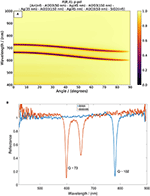Figure 1. (A) Reflectance of an IMIMIMI stack integrating two identical Fabry-Pérot cavities made of silver and aluminum oxide layers. The substrate is silicon dioxide and the first medium is air. (B) Experimental reflectance at normal illumination of two samples with single and double Fabry-Pérot cavities, indicating the respective Q-factor.

In Figure 2 are presented calculated and experimental results of the dispersion for two an three coupled cavities. In the calculated results (Figures 2A,B) the first and the last medium are the same. In the experimental results the illumination if from air and the substrate is glass. In the three coupled cavities there are two anti-crossings. The effect of the variation of the refrative index of the first medium is illustrated in Figure 3. For angles above 30° the lower curve deflects rapidly with an increase of the refractive index n1.

FIGURE 2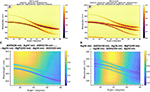Figure 2. Strong coupling in coupled planar Fabry-Pérot cavities. (A) Reflectance for two coupled cavities with different intra-cavity dielectric materials: aluminum oxide and magnesium fluoride. The strength of the coupling depends on the thickness of the inter-cavity silver layer. The tuning parameter to achieve the classical strong coupling is the angle on incidence of a plane wave. The dispersion also depends on refractive index of the first and the last medium. However, at vertical incidence the position of the resonances is almost unperturbed by changing the refractive index of the first medium. (B) Reflectance for 3 cavities. (C) Experimental reflectance for two cavities with p-polarized light illumination from air. (D) Experimental reflectance for three cavities in symmetric arrangement, where the middle cavity has the lower refractive index (MgF2).

FIGURE 3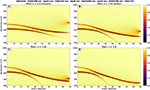Figure 3. Reflectance of coupled planar cavities presenting strong coupling with dependence on the refractive index of the first medium. At vertical incidence (θ = 0) the reflectance is independent of the refractive index of the first medium, but for angles larger then 30° fast deflection of the lower curve arises.

### 4.1. Guided Modes in Plasmonic-Dielectric Layered Media

The cavity modes discussed in the previous section are excited by propagating waves. Evanescent wave modes also exist for coupled Fabry-Pérot cavities. They propagate along any direction in the plane of the layers with constant β. For single and double metals layers forming IMI and IMIMI structures analytic and closed form solutions of the dispersion relation of surface plasmons exist (Economou, 1969; Raether, 1988). In structures with more layers of dispersive materials, the roots of the complex equations are difficult to obtain and other semi-analytical methods are more convenient.

The calculation of the dispersion of surface plasmon is done using two alternative methods. The first is based on the transfer matrix method, using a point dipole source located near the first dielectric-metal. The power density spectrum of the light radiated by the dipole is either transmitted through the stack, absorbed by the materials, or coupled into guided modes. The theory of the power loss of a dipole near a layered medium was developed by several researchers in the 70's and 80's of the last century (Chance et al., 1978; Sipe, 1981; Ford and Weber, 1984) and applied in problems of radiative decay engineering of fluorescent dyes near interfaces (Barnes, 1998, 1999; Novotny and Hecht, 2012).

The dispersion of plasmonic modes is achieved by evaluation of the integrand functions of the power loss of a harmonic oscillating dipole very close to a metal surface. According to the semi-classical theory, the decay rate of a dipole in a homogeneous medium of dielectric permittivity ϵ1 is given by γ = ${P}$/(ℏω), with

where μ is the dipole moment. Near an interface separating the medium where the dipole is located from other semi-infinite medium, or layered media, the power loss is dependent on the reflection coefficients of these media and the distance to the dipole. There is a radiating contribution and a non-radiating contribution to the decay rate, depending on the k-vector of the radiating field. The radiating contribution to the power spectrum is given by

where k1 is the k-vector of the medium where the dipole is located. The non-radiating contribution includes guided modes, namely surface plasmon modes associated with non-propagating k-vectors larger than k1. These modes can be calculated based on the Fresnel reflection coefficients from the boundary for the s- and p-polarization. The quantum efficiency of the radiation is given by the ratio

The decay rate can be decomposed into a parallel (in-plane) and a perpendicular (out-of-plane) term. They affect differently the decay rate, because they rely on different contributions of the Fresnel reflection coefficients for p- and s-polarization. The density of power loss of an isotropic dipole, as it is expected from a dye molecule embedded in a dielectric transparent medium, is given by

For a dipole near in a medium of dielectric permittivity ϵ1 at a distance d to a second medium of permittivity ϵ2 (or an arbitrary layered medium) the decay rate for the parallel and perpendicular components is given by Ford and Weber (1984) and Barnes (1998)

where

and k0 = ω/c, u = k1/k0 and ${l}_{i}=-i{\left({ϵ}_{j}/{ϵ}_{1}-{u}^{2}\right)}^{1/2}$ with j = 1, 2.

However, in order to obtain the dispersion relation these integrals have not to be evaluated. If the second medium is substituted by a layered medium of dielectric and plasmonic layers, the corresponding reflection coefficients are obtained from the transfer matrix calculation.

The second method to obtain the dispersion relation relies on the scattering matrix calculation. It is a semi-analytical calculation method. The main advantage of the scattering matrix comparing to the transfer matrix is its numerical stability, in particular when absorbing media are involved. The dispersion is found by solving the roots of the following equation, by the steepest descent method (Defrance et al., 2016)

and f is determined using the scattering matrix for the layered structure. The MATLAB library MOOSH was employed for this purpose (Defrance et al., 2016).

The dispersion of the IMIMI stack calculated using the scattering matrix formalism is presented in Figure 4. The density of power loss of an isotropic dipole localized 5 nm above the first silver layer is presented in Figure 5. Both dispersions relations are equivalent, but the normalized k-vector is different. Hence, the differences in the values of the modes in the absissa. In Figure 4 kx was normalized by the vacuum k0, whereas in Figure 5 kx the normalization constant is k1 = k0n1. The multiple plasmon modes arise as result of a hybridization between the anti-symmetric and the symmetric surface plasmon modes of a single film and the cavity mode between the metal layers. They form two branches: the lower energy branch includes both fundamental modes and a number of modes of larger β identical to the number of coupled cavities. For a single Fabry-Pérot cavity is one. At higher energy levels the second branch has two modes, the anti-symmetric mode and a volume mode. Both branches converge to a flat dispersion for large propagation constant around 3 eV (see Figure 5). The modes splitting and saturation for long propagation constant is a feature shared by layered hyperbolic metamaterials (Cortes et al., 2012; Shekhar and Jacob, 2014), discussed in the next section. The upper branch corresponds to a Type I hyperbolic metamaterial and the lower branch of modes corresponds to Type II, respectively (Cortes et al., 2012; Shekhar and Jacob, 2014). The plasmon modes of large k-vector propagate inside the layered medium and are therefore called volume plasmons.

FIGURE 4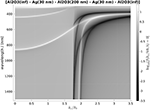Figure 4. Dispersion relation of the surface plasmon modes supported in a pair of silver films separated by a layer of aluminum oxide. The semi-infinite media embedding the three layers is also aluminum oxide. The dielectric constants for silver were taken from Johnson and Christy (1972). The dielectric function used for the aluminum oxide was based on the Sellmeier dispersion equation found by Malitson (1962). The tabulated optical constant values were extracted from the online database https://refractiveindex.info (Polyanskiy, 2020). The dark regions correspond to the plasmon guided modes. Plasmon modes have always a k-vector larger then k1 = k0n1, the propagation constant of the first medium. The bright lines in the left region denote the cavity modes for p-polarized light.

FIGURE 5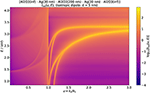Figure 5. Dispersion relation of the SPP modes supported in a pair of silver films separated by a dielectric layer of aluminum oxide. The materials and geometrical parameters are the same of the Figure 4. The bright regions correspond to the highest density of power loss of an isotropic dipole located at 5 nm away the first silver surface. The less pronounced curves for effective index kx/k0 < 1.0, correspond to TM and TE cavity modes.

Samples were fabricated using bilayers of Al2O3 and silver with thickness d ≪ λ. The experimental investigation of these samples, namely the properties of propagating plasmons and the fluorescence lifetime of emitters near the first silver layer can provide more information for their potential application in quantum optics experiments.

## 5. Hyperbolic Metamaterials

A hyperbolic metamaterial is a material which has a dispersion relation described by a hyperbolic equation (Shekhar et al., 2014). The isofrequency surfaces of isotropic materials have spherical shape. When a dielectric material is birefringent in a single axial direction it is called uniaxial and its dispersion equation has isofrequency surfaces of spheroidal shape, whereas biaxial materials have ellipsoidal isofrequency surfaces. The effective medium theory (EMT) (Bruggeman, 1935; Rytov, 1956) is a method of averaging the dielectric function according to the symmetry axes of the lattice. An early application of the EMT goes back to investigation of the colors of colloidal particles in glasses (Garnett, 1904). The homogenization of the EMT requires a unit cell size much smaller than the wavelength of light in the frequency range considered. The resulting dielectric function is calculated based on geometrical parameters and on the dielectric functions of the constituent materials and their lengths along the symmetry axes of the periodic structure. For a layered medium made of binary layers of materials with dielectric functions ϵ1(ω) and ϵ2(ω) and thicknesses d1 and d2, respectively, the EMT dielectric function results into two components: one for any axis parallel to the layers and one for the axis perpendicular to the layers. Assuming that the layers are parallel to XY-plane, then ϵxx = ϵyy = ϵ and ϵzz = ϵ and using k0 = ω/c the in-plane and the out-of-plane dielectric functions read

The corresponding dispersion equation takes the form (Guo et al., 2020)

The first term corresponds to the ordinary TE wave and the corresponding isofrequency surfaces (ω = const.) are spherical. The second term corresponds to the extraordinary TM waves. When ϵ > 0 and ϵ < 0 both modes exist and the second term leads to a dispersion whose isofrequency have double sheet hyperboloid shape (Type I). For ϵ < 0 and ϵ > 0 only the TM waves can propagate and isofrequency surfaces have single sheet hyperboloid shape (Type II). Type II hyperboloids have negative Gaussian curvature everywhere, whereas Type I hyperboloids have positive Gaussian curvature.

A hyperbolic material of Type II dispersion requires one constituent layer allowing large anisotropy, for example a material of negative dispersion (plasmonic). By other hand, an array of dielectric rods embedded in a distinct dielectric medium achieve a EMT dielectric function made of two parts: a spheroidal dielectric function for small k-vectors and a Type I hyperboloidal function for large k-vectors (Shekhar et al., 2014). However, according to the EMT a transition between Type II and type I can be achieved, depending on the filling factor of the plasmonic material in the unit cell and on the wavelength range (Cortes et al., 2012).

Array of rods of dielectric function ϵm(ω) embedded in a isotropic medium of dielectric function ϵd form other example of hyperbolic metamaterial, where the EMT homogenization can also be applied. Rods of diameter r and lattice constant L arranged in a squared lattice and oriented along the z-axis have the following EMT dielectric functions (Shekhar et al., 2014):

The filling factor ρ = a/A, is defined as the ratio between the area of the cross-section of the rods a and the area of the unit cell of the lattice A.

The effective dielectric function components of a hyperbolic material made of an infinite number of silver (10 nm thickness) and Al2O3 (20 nm) layers are presented in Figure 6. The parallel and perpendicular components were calculated using the effective medium theory equations.

FIGURE 6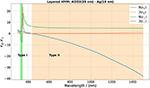Figure 6. Dielectric functions of a plasmonic-dielectric layered medium calculated using the effective medium theory. Each bilayer is composed of silver (10 nm) and aluminum oxide (20 nm). The permittivity of silver was obtained from the optical constants from Johnson and Christy (1972). The spectral regions labeled with Type I and Type II are the regions where the real part of perpendicular and parallel parts of the dielectric function become negative, respectively.

### 5.1. Bulk Plasmon Modes in Finite Hyperbolic Stacks

In order to avoid the limitations of the effective medium theory (Kidwai et al., 2012) and the Kronig-Penney model for infinite number of layers (Li and Khurgin, 2016) the calculations of the field profiles in layered media of finite length were based on the scattering matrix method. Magnetic field profiles of surface modes and the bulk plasmon modes for two finite stacks of dielectric and metal layers are presented in Figure 7 and Figure 8. Both structures have bilayers of the same thickness. The difference is the number silver films: 5 in Figure 7 and 6 in Figure 8. The wavelengths at which the profiles were calculated are in the range of the Type II hyperbolic modes branch.

FIGURE 7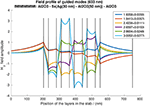Figure 7. Profiles of the field component Hy for λ = 633 nm along the stack of 5 silver layers of 30 nm thickness, separated by aluminum oxide layers of 50 nm thickness. The first two lines correspond to the Hy with symmetric and antisymmetric profile, respectively. The two modes have maximum amplitude at the external boundaries. By contrary, the bulk modes have increasing amplitudes with increasing kr. The vertical black lines indicate the boundaries between layers.

FIGURE 8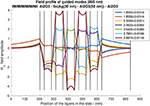Figure 8. Profiles of the field component Hy for λ = 800 nm along the stack of 6 silver layers of 30 nm thickness, separated by aluminum oxide layers of 50 nm thickness. The vertical black lines indicate the boundaries between layers. The symmetry center is in the central dielectric layer.

The following properties can be inferred from the profiles of the Hy field component: (a) the first mode with a symmetric profile of the magnetic field along the full structure has the smallest k-vector (normalized to k0). It corresponds to the antisymmetric electric field mode. (b) The second mode with a slightly larger propagation constant has an antisymmetric magnetic field distribution, considering the symmetry plane of the full structure. (c) The volume plasmon modes have successive increasing values of the propagation constants and simultaneously increasing amplitudes inside the layers. (d) The imaginary part of the propagation constant ${J}$[β] = ki increases with the order of the mode and the respective real part kr. This means that the propagation length of the bulk plasmons decreases with an increase of the propagation constant. Therefore, an arbitrary large number of layers and modes may be counterproductive in applications as imaging (Li et al., 2017) and long-range interactions (Biehs et al., 2016; Newman et al., 2018). Due to the large field strength inside some layers and the well-separated propagation constants of the volume modes it would be of high relevance the selective excitation of single plasmon modes.

## 6. Photonic Topological Materials in One-Dimension

The emergence of the topological order in condensed matter physics has revolutionize materials science, namely in its solid state and optical domains. Starting with the fractional quantum Hall effect and the quantum spin Hall effect, where the electron current is replaced by a spin current, an increasing number of effects has been discovered to posses topological properties as the topological insulators (Hasan and Kane, 2010). Moreover, the concepts of topological phase transition and topological insulator have been also extended into photonics (Ozawa et al., 2019).

It is also noteworthy that an early discovery of electron states in truncated crystals, the edge states, are now investigated from the perspective of topological insulators, giving them a much large degree of generality. The impact of topological materials and topological insulators has achieved a large scale attraction, from effects arising in classical waves to states of quantum matter (Hasan and Kane, 2010; Haldane, 2017). Topology properties are more general than geometry properties as symmetry. A topological space preserves its properties by a continuous deformation, whereas an arbitrary space deformation often means a break of symmetry. Thus, properties of physical systems with topological invariance are robust against small deformations and defects.

One of the most relevant examples of topological properties is the Berry phase (Berry, 1984). It generalizes the concept of geometric phase introduced by Pancharatnam (1956) and it is related to the parallel transport in topological spaces. The close relationship of the Berry phase, arising in a cyclic path of a quantum system described by a Hamiltonian under an adiabatic evolution, with topological properties as the Chern number and other topological invariants arising in condensed matter physics was due to Simon (1983). It is remarkable that the publication date of the article of B. Simon preceded that of the article of M. Berry and coined the name of this phase. Reviews of phenomena in condensed matter physics manifesting Berry phase are available (Resta, 2000; Xiao et al., 2010).

Zak investigated the application to the Berry phase is solid state materials characterized by a periodic lattice in real space and by the corresponding Brillouin zone (BZ) in the momentum space (Zak, 1989). Due to the periodicity of BZ the evolution of a Bloch wave along a band of the BZ is equivalent to a closed loop. Zak also found that bands of a periodic lattice, in particular in one-dimension, manifest certain symmetry properties when crossing. These properties are required for the generation of edge states (Zak, 1984, 1985).

The most well-known examples of edge states of electrons in solids are the Tamm states (Tamm, 1932a,b) and Schockley states (Shockley, 1939). Both are variants of the same kind of edges states (Vinogradov et al., 2010). The advent of photonic crystals brought out the investigation of the optical analogues of the edge states known for electrons in solids (Stȩślicka et al., 1990; Tikhodeev, 1991; Kavokin et al., 2005; Kaliteevski et al., 2007; Vinogradov et al., 2010) as well as in two-dimensional materials (Nakada et al., 1996; Delplace et al., 2011). Also related to formation of edge states in one-dimension is the model of Su-Schrieffer-Heeger describing the excitation of solitons in polyacetylene (Su et al., 1979) and the Peierls transition. The electron edges states of one-dimensional atom lattices have an analog in photonics, substituting the periodic potential by a layered structure with periodic refractive index.

Topological invariants of the photonic band structure in photonic crystals and in plasmonic materials coupled with photonic crystals, as the Zak phase play an important role in the edge state properties (Xiao et al., 2014, 2015; Gao et al., 2015; Henriques et al., 2020). Moreover, a requirement for the generation of edge states in crystals is the band inversion (Zak, 1989; Xiao et al., 2014, 2015; Esmann et al., 2018; Wang et al., 2019). Despite the fact that the edge states of photonic crystals are only analogs of the edge electronic states discovered by Tamm, for historical reasons it is reasonable the call edge states arising in truncated photonic crystals optical Tamm states (OTS).

Presently many publications are dedicated to the study of edge states and the associated topological properties in two- and three-dimensional photonic crystals and in arrays of plasmonic particles (Wang et al., 2008, 2016; van Miert et al., 2016; Proctor et al., 2020). In this article only one-dimensional edge states and their properties are discussed.

### 6.1. Design of Single and Multiple Optical Tamm States

Up to date most of the optical Tamm states investigated are based either on structures made of two planar photonic crystals (Kavokin et al., 2005; Vinogradov et al., 2010; Xiao et al., 2014; Esmann et al., 2018), where one of them presents band inversion, or structures based on a photonic crystal and plasmonic materials (Durach and Rusina, 2012; Henriques et al., 2020), or an excitonic material (Núñez-Sánchez et al., 2016). Moreover, the analogy between photonic layered media and electronic lattices has been also extended to systems supporting mechanical waves (Xiao et al., 2015; Yang et al., 2016; Ma et al., 2019).

The design of edge states arising in stacks of photonic crystals relies on the definition of a bulk bandgap, in an infinite photonic crystal (PC1), and by establishing a correspondence between the bulk and the edge state. This requires that the second photonic crystal (PC2) should have an overlapping bandgap with the first after operating a band inversion. This excludes the second photonic crystal to be identical to the first. In photonic crystals with binary layers the band inversion can be achieved by changing either the values of refractive indices of the materials of the layers, or by changing their thicknesses. Using the commercial available oxides with transparency in the visible and NIR spectral regions, it is more practical to change the thickness of each layer. Semiconductors of different materials can also be used either in phononic Tamm states (Esmann et al., 2018), or in electronic Tamm states in superlattices (Steslicka, 1995). The band inversion of one-dimensional photonic crystals is the same kind of band transformation in two-dimensional crystals, in order to obtain topological insulators (Parappurath et al., 2020).

In a photonic crystal of indefinite length the bulk Bloch modes (bands) for illumination at vertical incidence (θ = 0) are found from the dispersion relation of the layered medium, which reads (Yeh, 2005)

with k1 = (ω/c)n1 and k2 = (ω/c)n2. The thicknesses of the layers are d1 = a and d2 = b and the refractive indices n1 and n2, respectively. KB is the Bloch k-vector and Λ = a + b. A similar equation arises in the dispersion of the Kronig-Penney model of electrons in solids (Kronig and Penney, 1931). The same method was used by I. Tamm to find the edge states of electrons in a one-dimensional lattice, but substituting the propagation constants k1 and k2 by constants related to the periodic potential function.

Forbidden gaps in the energy occur for ${R}$[KB] = /Λ, with n = 1, 2, … . In the forbidden bands KBΛ = π ± ix, where x is a function of the refractive indices n1 and n2 (Yeh, 2005). Bandgaps centered in the wavelength λ = λg arise when the following condition is verified

or equivalently,

The band edges are also dependent on n1 and n2. They determine the width of each bandgap. By varying a and b opening or closing of bandgaps can be achieved. When two semi-infinite photonic crystals are stacked together and truncated at the same inversion point of the unit cell of the lattice, with two forbidden bands overlap, but the Zak phases of the respective edge bands reverses an edge state arises. This permits total transmission at a very narrow bandwidth inside the bandgap. The tuning of the parameters of the gaps and the respective Zak phases was discussed in detail in Xiao et al. (2014). I should be noted that the definition of the unit cell centered around an inversion point is necessary for a well-defined value of the Zak phase (0 oi π), but it is not a requirement for the generation of an edge state.

Using the treatment of optical impedance leads to the conditions necessary to achieve full transmission inside the gap. This is Z1 + Z2 = 0 where Z1 and Z2 are the input optical impedances of the first and the second semi-infinite crystals, respectively. Konopsky found analytic solutions for input impedance for s- and p-polarized light combining photonic crystals and dispersive media (Konopsky, 2010). The formulas obtained permit application in quite general situations accounting for angular dependence of the incoming wave. The estimation of optimal conditions of excitation on LRSPP in planar layered media also benefit from this calculation method (Konopsky and Alieva, 2009; Delfan et al., 2015).

Despite the simplicity of the impedance condition, the practical design of optical Tamm states for an arbitrary forbidden band centered around λg is more cumbersome. In practice we deal with finite crystals where the Kronig-Penney model cannot be applied. A practical way to tailor the thickness of the layers for which the edge states could be found is based on the phase of the reflection coefficient at vertical incidence (Kavokin et al., 2005; Xiao et al., 2014; Gao et al., 2015). For that we calculate the total reflection coefficient of a finite binary photonic crystal using the transfer matrix method. The phase of the reflection coefficient can be made dependent on a parameter δ defined in the following way. We set the central wavelength of the forbidden band to be λg and determine the quarter wavelength thicknesses to be a0 and b0 for the higher and lower refractive index layers, respectively. The reflectance and transmittance and respective reflection and transmission coefficients, as the phase of the reflection coefficient can be obtained for any combination of a and b such that a = a0(1 + δ) and b = b0(1 − δ), with −1 < δ < 1. Thus, the extreme pairs are a = 2a0 and b = 0, or a = 0 and b = 2b0.

In Figure 9 are shown results of the transmittance and phase of the reflection coefficient of a photonic crystal made of SiO2 and ZrO2 with 7 units cells. a0 and b0 were estimated for two central wavelengths λg = 600 nm and λg = 1, 200 nm. The unit cell is constituted by three layers: two lateral layers of thickness a/2 = λg/(nh8) and a central layer of thickness b = λg/(nl4). Therefore, the total optical length is λg/2. A unit cell built by this way has a mirror symmetry (inversion center) at the middle of the layer of lower refractive index and is required for a well-defined value of the Zak phase in the dispersion bands. Arbitrary values of the thickness of each layer, keeping the total optical length constant, would not change the forbidden band for an infinite crystal but change the symmetry properties of the bands in the dispersion relation (Zak, 1984; Xiao et al., 2014). The forbidden bands are the dark regions in the transmittance (Figure 9). The corresponding phase of the reflection coefficient exhibit gradients either positive from 0 to π, or negative from 0 to −π. Outside the forbidden bands the average phase is large and changes abruptly from π to −π.

FIGURE 9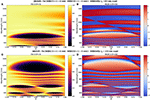Figure 9. Transmittance, (A,C), and phase of the reflection coefficient, (B,D), of photonic crystals with 7 unit cells on a silica substrate. In the upper row the thickness of the layer with higher refractive index is a = a0(1 + δ) nm and the layer with lower refractive index has a thickness b = b0(1 − δ). The initial values of layers are a0 = 70 nm and b0 = 100 nm, respectively. In the lower row the values are a0 = 135 nm and b0 = 200 nm. The choice of the initial thickness of each layer was determined by the central wavelength and the first bandgap. λ = 600 nm in the upper row and λ = 1, 200 nm in the lower row. The parameter δ varies between –1 and 1. The tuning of optical Tamm states is achieved by combining two photonic crystals with layers thickness corresponding to overlapping bandgaps in energy and symmetric gradients (blue and red) of the phase of the reflection coefficient at a predefined wavelength.

By stacking two or more finite crystals (see Figure 10) with alternating sign of the phase of the reflection coefficient, edge states arise when the impedances match. The number of solutions of the impedance condition is not necessarily single valued. Indeed multiple edge states can be achieved. Two examples of edge states generated at the first bandgap of quarter wavelength crystals and their counterparts with band inversion are presented in Figure 11. In a stack of two photonic crystals PC1-PC2 using the same materials, where the dimensions of the PC2 were tailored in order that its second band gap has opposite sign of the reflection coefficient, only ore edge state arises (Figure 11A). This optical Tamm state results in a narrow transmission line in the middle of bandgap. The wavelength of this state at vertical illumination differs from λg = 600 nm, due to the small variation of thickness of the layers and the wavelength dependence of the dielectric functions, not considered in the optical length equation.

FIGURE 10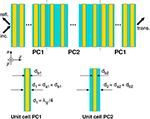Figure 10. Schematic representation of a stack based on two photonic crystals of different unit cells. The PC2 is designed in order to have a bandgap overlapping with the first bandgap of PC1, after a band inversion. Multiple edge states are formed by attaching alternately PC1 and PC2 crystals.

FIGURE 11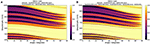Figure 11. Reflectance of stacks of two (A) and three (B) photonic crystals producing optical Tamm states in the bandgap, as function of the angle of incidence. The incidence of s-polarized light is from air and the substrate of the stack is silica. The optical Tamm states correspond to full transmission lines in the forbidden band, indicated by the narrow dark curves in the center of the bandgap. In (A) there is only one line. In (B), the interaction between symmetric edge states produces 4 lines.

When three PCs are stacked together, the edge states at both boundaries can couple and lead to double transmission lines (Figure 11B). Surprisingly, not only two lines appear but 4. The central transmission narrow bands are expected from the frequency splitting due the coupling between edge states of both boundaries between PC1 and PC2. They are located at almost identical distance to the wavelength of the single edge state. But other two narrow bands arise at larger distance approaching the edges of the bandgap. The full-width at half-maximum (FWHM) of the narrow bands is typically smaller than 0.5 nm for the PCs with 7 unit cells, but decreases rapidly with increasing number of units cells. Therefore, it is worth to look more in detail into the field profiles and respective phase for the edge states in a PC1-PC2-PC1 stack (Figures 12, 13). The field component Ey (corresponding to s-polarized light at vertical incidence) and its modulus along the transverse direction of stack indicates the type of edge state that is formed. The field inside the forbidden band decay exponentially with the distance, characteristic of an evanescent wave. Thus, the reflection coefficient can be expressed by r = 1.0 exp(). Its phase has either a positive or negative value. At the edge states the field distribution has a fully distinct pattern inside the structure, peaking at the boundary of the PCs and decaying with z in both directions (Figure 13A). The number of unit cells of the PC2 determines the strength of the coupling and the corresponding frequency splitting width. Therefore, at large number of unit cells the edge state do not interact and both spectral lines degenerate into the single line characteristic of a single edge state. The smallest thickness of the PC2 is a single unit cell, for which the interaction between edge states and the separation between the corresponding transmission lines is maximum.

FIGURE 12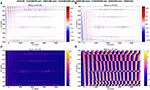Figure 12. Optical Tamm states in a symmetric structure constituted by 3 one-dimensional photonic crystals. The unit cell of the first and the third PCs is made of layers with quarter wavelength thickness. The unit cell is built by a central layer of lower refractive index nl and thickness of λ/(4nl), surrounded by two layers of thickness λ/(8nh) of a material with higher refractive index. The wavelength λ is selected to be central wavelength of the first bandgap. In the central PC, the thickness of the layers of low refractive index can remain the same, whereas the thickness of the layer of high refractive index is increased in order to achieve a band inversion. In the bandgap the amplitude of the fields decays rapidly, except at the wavelengths corresponding to edge states. (A) real part, (B) imaginary part, (C) modulus and (D) phase of the electric field across the photonic crystal structure. The optical Tamm states are in the middle of the band gap.

FIGURE 13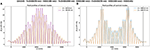Figure 13. Profiles of |Eyi, z)| for the 4 narrow bands in the bandgap. The central OTS have maxima at the boundaries of the photonic crystals and decay in booth directions with the distance. Their profiles are almost symmetric and overlap in the both external PCs, but not in the central PC2. The profiles of the lateral modes have maxima localized in the central PC2 and decay in both directions. Profiles of the modulus of the electric field along the propagation axis for the lateral modes (A) and central modes (optical Tamm states) (B).

The field profiles of the lateral spectral lines in the bandgap that arise in the symmetric structure PC1-PC2-PC1 cannot be explained by a equivalence between interacting edge states and narrow transmitted lines. The field distribution has maxima centered in the stack and not at the PCs boundary. However, they have similar narrow bandwidth and appear independently of the thickness of PC2. More intriguingly, the number of lateral bands inside the bandgap increases slowly with the number of unit cells of PC2. This is the behavior expected for a Fabry-Pérot resonator, with the difference that the lateral modes here occur in both sides of the central OTS modes. The resonance bandwidth of the optical Tamm state modes is comparable to that of Fabry-Pérot resonators based on identical Bragg mirrors of the same length. However, the relative distance of the resonances in the forbidden band to the band edges remains almost constant, when the angle of incidence varies from 0° to 90° (see Figure 11). By contrast, in a Fabry-Pérot resonator the cavity resonances tend to cross the forbidden band as function of the angle of incidence, while the free spectral range remains constant (Saleh and Teich, 2007).

The formation of multiple edge states based on binary and quaternary units cells was investigated in Bianchi and Kahn (2020). The interplaying between Fabry-Pérot modes and optical Tamm states in structure formed by a photonic crystal and two metallic mirrors was studied in Durach and Rusina (2012). A deeper analysis of the OTS and lateral modes arising in multiple alternating PCs with band inversion and between PCs and plasmomic layers is outside the scope of the present article and will be addressed in other publication.

In Figure 14 are presented experimental results of the reflectance on two samples measured at quasi-vertical incidence, using an objective of small numerical aperture for illumination and light collection (NA = 0.12). The samples were fabricated by alternated deposition of layers of Ta2O5 and SiO2 making a stack of three photonic crystals with 7, 3, and 7 units cells, respectively. The layers of the first and third PC have a nominal thickness of 70 nm (Ta2O5) and 100 nm (SiO2). The nominal thickness of the layers of the central PC are 200 nm (Ta2O5) and 100 nm (SiO2). The spectral shift of the bandgap and resonances are due to fabrication. Small changes in the effective layer thickness are due to different distances from each substrate to the crucible and different evaporation angle. Despite the limited conditions of the deposition pronounced dips corresponding to the OTS and lateral states are visible in the reflectance spectrum. The reflectance dips are probably deeper that measured, because the spectrometer used has a spectral resolution of approximately 5 nm.

FIGURE 14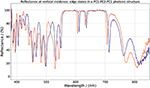Figure 14. Experimental reflectance presenting 4 narrow reflectance dips in the middle and at the edges of the bandgap. These dips correspond to optical Tamm states in the symmetric structure constituted by 3 photonic crystals. The first and third photonic crystals have 7 unit cells of Ta2O5 and SiO2 of quarter wavelength thickness, respectively. The central PC has 3 unit cells of the same materials with nominal thickness of 200 and 100 nm, respectively. Differences between theoretical and effective values of the thickness of each layer depend on the evaporation conditions and are responsible for shifts in the bandgap and position of the resonances.

When the central protonic crystal (PC2) of the former layered structure is substituted by a homogeneous layer of a material with refractive index n and thickness d ≤ λ/(2n) the ordinary Fabry-Pérot resonator is obtained. It must be stressed that in the structure PC1-PC2 the thickness of the layer containing the boundary of the crystals is d12 = (a1/2 + a2/2). Using the thickness values of the structure used in Figure 12A we have d12 = 135 nm. The material of thickness d12 is ZrO2 with a refractive index of n ≈ 2.16 at λ = 600 nm. This is approximately half wavelength in the medium for a vacuum wavelength of 600 nm. Thus, the single OTS can be regarded as the single Fabry-Pérot mode in cavity of refractive index nh and length d12. Indeed, using the wavelength of the OTS line of Figure 12A, λOTS ≈ 584 nm at vertical incidence, d12 = 135 nm is exactly half wavelength in ZrO2 of vacuum wavelength λ = 584 nm.

We can as well substitute the central PC2 by a plasmonic layer, as silver or gold. It is well-known that a stack of a quarter wavelength photonic crystal attached to a plasmonic layer produces an optical Tamm state (Vinogradov et al., 2006; Kaliteevski et al., 2007; Henriques et al., 2020). We can expect a double OTS for a thin layer of few tens of nanometer of silver at optical wavelengths. Effectively, two modes arise for a PC made of ZrO2, or Ta2O5 and SiO2. In Figure 15 are presented the reflectance as function of angle on incidence and the profile of the the electric field in the structure for excitation at vertical incidence. The spectral separation of the modes depends on the thickness of the silver layer, decreasing with increasing thickness. The symmetry properties of the plasmon modes are reflected in the field distribution of the edge states inside the bandgap (see Figure 16). The higher energy mode (λa = λ1 = 540.3 nm) has an anti-symmetry electric field distribution, using as reference the center of the silver layer. The mode with lower energy (λs = λ2 = 592.3 nm) has smaller amplitude and is symmetric. Hence, the symmetric and anti-symmetric modes of a plasmonic thin film are transformed inot a pair of optical Tamm states width symmetric and antisymmetric electric field distribution in the stack. Unlike in the symmetric configuration of single plasmonic film requiring evanescent wave excitation, the excitation of the both optical Tamm states is here from air, whereas a the substrate is silica.

FIGURE 15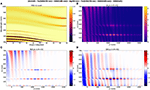Figure 15. Optical Tamm states in a symmetric structure constituted by two identical 7 unit cells photonic crystals and a central thin silver layer (dAg = 30 nm). The layers thickness in the unit cell of both PCs is approximately a quarter of the wavelength. Reflectance (A), |Ey(z, λ, θ = 0)|(B) and real (C) and imaginary (D) parts of Ey.

FIGURE 16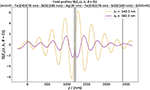Figure 16. Profiles of Ey along the z-axis in the stack for vertical incidence of light. The vertical lines indicate the boundaries of the photonic crystals and the silver layer.

## 7. Conclusions

In this article three types of resonances with narrow bandwidth involving layered plasmonic media were analyzed: resonances arising in coupled Fabry-Pérot cavities of silver mirrors with heterogeneous intra-cavity media, presenting optical cavity strong coupling; hyperbolic layered media based on sub-wavelength layers of plasmonic and dielectric films, supporting bulk plasmon modes with large propagation constant and large field amplitudes inside the structure; and finally, multiple optical Tamm states, arising in stacks of photonic crystals and in plasmonic films attached to photonic crystals.

The resonance anti-crossing in coupled Fabry-Pérot cavities is not only one of the most simple examples of classical strong coupling but also represents a platform with potential in refractive index sensing applications. Fabry-Pérot cavities based on distributed Bragg reflectors, instead of silver mirrors, could also be used for the strong coupling, but the total thickness of the stack is much larger and the sensitivity to variations of refractive index of external media much weaker.

The migration of Fabry-Pérot cavities into layered hyperbolic metamaterials is put straightforward. The analysis of the bulk plasmon modes inside layered hyperbolic metamaterials of finite size reveals two fundamental properties: the amplitude of the field increases with the real part of the propagation constant, and the value of the imaginary part ki also increases in the same order, which implies a systematic decrease of the plasmon propagation length. Thus, in applications requiring plasmons of long propagation constant, extremely thin layers in large number is counterproductive. A small number of bilayers supporting fewer plasmon modes could achieve better results in terms of averaged length of propagation. On the other hand, the increasing field amplitude with the plasmon mode order inside the stack can benefit applications based on nonlinear effects (Davoyan et al., 2009). The periodic plasmonic-dielectric multilayers can also be applied in fluorescence lifetime engineering. Excited nanoemitters on top of the stack can couple to the evanescent modes of the bulk plasmons. Fast fluorescence decay can be achieved due to the large density of optical modes in the stack. The strong near-field confinement allowed by the surface plasmons is related to the large progation constants with relatively low damping of the evanescent waves and thus, permits the optical interaction between distant objects, as in the Förster resonance energy transfer (FRET) (Roth et al., 2018; Gonçalves et al., 2020).

Multiple optical Tamm states arising in ternary photonic crystals, or symmetric structures made of a plasmonic film and two identical Bragg reflectors form a new family of resonances relying on edge states with topological properties. The number of full or high transmission narrow resonances increases with the number of Tamm states. Two kinds of resonances were found: hybridized Tamm modes resulting from the interaction of single optical Tamm states at short distance and lateral modes with apparent Bragg field distribution. The bandwidth of the resonances calculated using the experimental dielectric functions of the materials is typically less than 0.4 nm, for photonic crystal with a length of 7 unit cells. This corresponds to Q-factors of the order of 1,000s in the visible spectrum. Higher values can be reached by increasing the number of unit cells in the external Bragg mirrors. Such narrow resonances can be of high interest in nanolasers and photonic switching systems, if their spectral position could be changed by variation the dielectric function of one of the materials employed. For some geometries one-way propagation can also be investigated by introducing materials with non-reciprocal properties in the structure as in (Khanikaev et al., 2009). Optical Tamm states present resonant modes with a bandwidth comparable to Fabry-Pérot modes, in cavities based on identical distributed Bragg mirrors, but their spectral dependence on the angle of incidence differ considerably. Multiple OTS modes based on ternary photonic crystal stacks can provide a robust alternative to optical filters based on Fabry-Pérot cavities. By other side, OTS modes arising in structures including plasmonic layers as the PC-Ag-PC, are also useful in sensing applications due to the narrow resonances, but can only be restricted to few plasmonic layers owing to the optical absorption. To my knowledge, the excitation of the two OTS modes with symmetric and antisymmetric field profiles was analyzed for the first time.

## Data Availability Statement

The raw data supporting the conclusions of this article will be made available by the authors, without undue reservation.

## Author Contributions

The author confirms being the sole contributor of this work and has approved it for publication.

## Funding

Some materials used in the preparation of samples were funded by the German Federal Ministry of Education and Research (BMBF) Grant 01DK17040.

## Conflict of Interest

The author declares that the research was conducted in the absence of any commercial or financial relationships that could be construed as a potential conflict of interest.

## Acknowledgments

The author thanks the Institute of Solid State Physics of the Ulm University for the evaporation facilities used in the fabrication of the layered structures and the technicians of the Institute of Experimental Physics for the preparation of substrates.

## Footnotes

1. ^The upper and lower media can be analyzed even more generally than dielectric and metal as in Yang et al. (1991).

## References

Abbott, B. P., Abbott, R., Abbott, T. D., Abernathy, M. R., Acernese, F., Ackley, K., et al. (2016). Observation of gravitational waves from a binary black hole merger. Phys. Rev. Lett. 116:061102. doi: 10.1103/PhysRevLett.116.061102

Ameling, R., and Giessen, H. (2012). Microcavity plasmonics: strong coupling of photonic cavities and plasmons. Laser Photon. Rev. 7, 141–169. doi: 10.1002/lpor.201100041

Amos, R. M., and Barnes, W. L. (1997). Modification of the spontaneous emission rate of Eu3+ ions close to a thin metal mirror. Phys. Rev. B 55, 7249–7254. doi: 10.1103/PhysRevB.55.7249

Barnes, W. L. (1998). Fluorescence near interfaces: the role of photonic mode density. J. Mod. Opt. 45, 661–699. doi: 10.1080/09500349808230614

Barnes, W. L. (1999). Electromagnetic crystals for surface plasmon polaritons and the extraction of light from emissive devices. J. Lightwave Technol. 17, 2170–2182. doi: 10.1109/50.803008

Bellessa, J., Bonnand, C., Plenet, J. C., and Mugnier, J. (2004). Strong coupling between surface plasmons and excitons in an organic semiconductor. Phys. Rev. Lett. 93:036404. doi: 10.1103/PhysRevLett.93.036404

Berini, P. (2009). Long-range surface plasmon polaritons. Adv. Opt. Photon. 1:484. doi: 10.1364/AOP.1.000484

Berini, P., Charbonneau, R., Jetté-Charbonneau, S., Lahoud, N., and Mattiussi, G. (2007). Long-range surface plasmon-polariton waveguides and devices in lithium niobate. J. Appl. Phys. 101:113114. doi: 10.1063/1.2739300

Berry, M. V. (1984). Quantal phase factors accompanying adiabatic changes. Proc. R. Soc. Lond. A Math. Phys. Sci. 392, 45–57. doi: 10.1098/rspa.1984.0023

Bianchi, N. J., and Kahn, L. M. (2020). Optical states in a 1D superlattice with multiple photonic crystal interfaces. J. Opt. 22:065101. doi: 10.1088/2040-8986/ab896c

Biehs, S.-A., Menon, V. M., and Agarwal, G. S. (2016). Long-range dipole-dipole interaction and anomalous Förster energy transfer across a hyperbolic metamaterial. Phys. Rev. B 93:245439. doi: 10.1103/PhysRevB.93.245439

Bright, T. J., Watjen, J. I., Zhang, Z. M., Muratore, C., Voevodin, A. A., Koukis, D. I., et al. (2013). Infrared optical properties of amorphous and nanocrystalline Ta2O5 thin films. J. Appl. Phys. 114:083515. doi: 10.1063/1.4819325

Bruggeman, D. A. G. (1935). Berechnung verschiedener physikalischer Konstanten von heterogenen Substanzen. I. Dielektrizitätskonstanten und Leitfähigkeiten der Mischkörper aus isotropen Substanzen. Ann. Phys. 416, 636–664. doi: 10.1002/andp.19354160705

Byrnes, S. J. (2019). Multilayer optical calculations. arXiv. arXiv:1603.02720

Chance, R. R., Prock, A., and Silbey, R. (1978). “Molecular fluorescence and energy transfer near interfaces,” in Advances in Chemical Physics, Vol. XXXVII (Hoboken, NJ: JohnWiley & Sons, Inc.), 1–65. doi: 10.1002/9780470142561.ch1

Chikkaraddy, R., de Nijs, B., Benz, F., Barrow, S. J., Scherman, O. A., Rosta, E., et al. (2016). Single-molecule strong coupling at room temperature in plasmonic nanocavities. Nature 535, 127–130. doi: 10.1038/nature17974

Cortes, C. L., Newman, W., Molesky, S., and Jacob, Z. (2012). Quantum nanophotonics using hyperbolic metamaterials. J. Opt. 14:063001. doi: 10.1088/2040-8978/14/6/063001

Davoyan, A. R., Shadrivov, I. V., and Kivshar, Y. S. (2009). Quadratic phase matching in nonlinear plasmonic nanoscale waveguides. Opt. Express 17:20063. doi: 10.1364/OE.17.020063

de Marcos, L. V. R., Larruquert, J. I., Méndez, J. A., and Aznárez, J. A. (2017). Self-consistent optical constants of MgF2, LaF3, and CeF3 films. Opt. Mater. Express 7:989. doi: 10.1364/OME.7.000989

Defrance, J., Lemaître, C., Ajib, R., Benedicto, J., Mallet, E., Pollès, R., et al. (2016). Moosh: a numerical Swiss Army knife for the optics of multilayers in Octave/Matlab. J. Open Res. Softw. 4:e13. doi: 10.5334/jors.100

Delfan, A., Degli-Eredi, I., and Sipe, J. E. (2015). Long-range surface plasmons in multilayer structures. J. Opt. Soc. Am. B 32:1615. doi: 10.1364/JOSAB.32.001615

Delplace, P., Ullmo, D., and Montambaux, G. (2011). Zak phase and the existence of edge states in graphene. Phys. Rev. B 84:195452. doi: 10.1103/PhysRevB.84.195452

DeVore, J. R. (1951). Refractive indices of rutile and sphalerite. J. Opt. Soc. Am. 41:416. doi: 10.1364/JOSA.41.000416

Dodge, M. J. (1984). Refractive properties of magnesium fluoride. Appl. Opt. 23:1980. doi: 10.1364/AO.23.001980

Durach, M., and Rusina, A. (2012). Transforming Fabry-Pérot resonances into a Tamm mode. Phys. Rev. B 86:235312. doi: 10.1103/PhysRevB.86.235312

Economou, E. N. (1969). Surface plasmons in thin films. Phys. Rev. 182, 539–554. doi: 10.1103/PhysRev.182.539

Esmann, M., Lamberti, F. R., Senellart, P., Favero, I., Krebs, O., Lanco, L., et al. (2018). Topological nanophononic states by band inversion. Phys. Rev. B 97:155422. doi: 10.1103/PhysRevB.97.155422

Ford, G. W., and Weber, W. H. (1984). Electromagnetic interactions of molecules with metal surfaces. Phys. Rep. 113, 195–287. doi: 10.1016/0370-1573(84)90098-X

Franke, E., Trimble, C. L., DeVries, M. J., Woollam, J. A., Schubert, M., and Frost, F. (2000). Dielectric function of amorphous tantalum oxide from the far infrared to the deep ultraviolet spectral region measured by spectroscopic ellipsometry. J. Appl. Phys. 88, 5166–5174. doi: 10.1063/1.1313784

Gao, L., Lemarchand, F., and Lequime, M. (2012). Exploitation of multiple incidences spectrometric measurements for thin film reverse engineering. Opt. Express 20:15734. doi: 10.1364/OE.20.015734

Gao, W. S., Xiao, M., Chan, C. T., and Tam, W. Y. (2015). Determination of Zak phase by reflection phase in 1D photonic crystals. Opt. Lett. 40:5259. doi: 10.1364/OL.40.005259

Garnett, J. C. M. (1904). XII. Colours in metal glasses and in metallic films. Philos. Trans. R. Soc. Lond. Ser. A 203, 385–420. doi: 10.1098/rsta.1904.0024

Gonçalves, P. A. D., Christensen, T., Rivera, N., Jauho, A.-P., Mortensen, N. A., and Soljačić, M. (2020). Plasmon-emitter interactions at the nanoscale. Nat. Commun. 11:366. doi: 10.1038/s41467-019-13820-z

Granata, M., Amato, A., Balzarini, L., Canepa, M., Degallaix, J., Forest, D., et al. (2020). Amorphous optical coatings of present gravitational-wave interferometers. Class. Quant. Gravity 37:095004. doi: 10.1088/1361-6382/ab77e9

Guo, Z., Jiang, H., and Chen, H. (2020). Hyperbolic metamaterials: from dispersion manipulation to applications. J. Appl. Phys. 127:071101. doi: 10.1063/1.5128679

Haldane, F. D. M. (2017). Nobel Lecture: topological quantum matter. Rev. Mod. Phys. 89:040502. doi: 10.1103/RevModPhys.89.040502

Hasan, M. Z., and Kane, C. L. (2010). Colloquium: topological insulators. Rev. Mod. Phys. 82, 3045–3067. doi: 10.1103/RevModPhys.82.3045

Henriques, J. C. G., Rappoport, T. G., Bludov, Y. V., Vasilevskiy, M. I., and Peres, N. M. R. (2020). Topological photonic Tamm states and the Su-Schrieffer-Heeger model. Phys. Rev. A 101:043811. doi: 10.1103/PhysRevA.101.043811

Homola, J. (2008). Surface plasmon resonance sensors for detection of chemical and biological species. Chem. Rev. 108, 462–493. doi: 10.1021/cr068107d

Johnson, P. B., and Christy, R. W. (1972). Optical constants of the noble metals. Phys. Rev. B 6, 4370–4379. doi: 10.1103/PhysRevB.6.4370

Kaliteevski, M., Iorsh, I., Brand, S., Abram, R. A., Chamberlain, J. M., Kavokin, A. V., et al. (2007). Tamm plasmon-polaritons: possible electromagnetic states at the interface of a metal and a dielectric Bragg mirror. Phys. Rev. B 76:165415. doi: 10.1103/PhysRevB.76.165415

Kavokin, A. V., Shelykh, I. A., and Malpuech, G. (2005). Lossless interface modes at the boundary between two periodic dielectric structures. Phys. Rev. B 72:233102. doi: 10.1103/PhysRevB.72.233102

Khanikaev, A. B., Baryshev, A. V., Inoue, M., and Kivshar, Y. S. (2009). One-way electromagnetic Tamm states in magnetophotonic structures. Appl. Phys. Lett. 95:011101. doi: 10.1063/1.3167356

Kidwai, O., Zhukovsky, S. V., and Sipe, J. E. (2012). Effective-medium approach to planar multilayer hyperbolic metamaterials: strengths and limitations. Phys. Rev. A 85:053842. doi: 10.1103/PhysRevA.85.053842

Ko, D. Y. K., and Inkson, J. C. (1988). Matrix method for tunneling in heterostructures: resonant tunneling in multilayer systems. Phys. Rev. B 38, 9945–9951. doi: 10.1103/PhysRevB.38.9945

Konopsky, V. N. (2010). Plasmon-polariton waves in nanofilms on one-dimensional photonic crystal surfaces. N. J. Phys. 12:093006. doi: 10.1088/1367-2630/12/9/093006

Konopsky, V. N., and Alieva, E. V. (2009). Long-range plasmons in lossy metal films on photonic crystal surfaces. Opt. Lett. 34:479. doi: 10.1364/OL.34.000479

Kretschmann, E., and Raether, H. (1968). Notizen: radiative decay of non radiative surface plasmons excited by light. Z. Naturforsch. A 23, 2135–2136. doi: 10.1515/zna-1968-1247

Kronig, R. D. L., and Penney, W. G. (1931). Quantum mechanics of electrons in crystal lattices. Proc. R. Soc. Lond. Ser. A 130, 499–513. doi: 10.1098/rspa.1931.0019

Li, L. (1996). Formulation and comparison of two recursive matrix algorithms for modeling layered diffraction gratings. J. Opt. Soc. Am. A 13:1024. doi: 10.1364/JOSAA.13.001024

Li, T., and Khurgin, J. B. (2016). Hyperbolic metamaterials: beyond the effective medium theory. Optica 3:1388. doi: 10.1364/OPTICA.3.001388

Li, T., Nagal, V., Gracias, D. H., and Khurgin, J. B. (2017). Limits of imaging with multilayer hyperbolic metamaterials. Opt. Express 25:13588. doi: 10.1364/OE.25.013588

Liebermann, T., and Knoll, W. (2000). Surface-plasmon field-enhanced fluorescence spectroscopy. Colloids Surf. A. Physicochem. Eng. Asp. 171, 115–130. doi: 10.1016/S0927-7757(99)00550-6

Ma, G., Xiao, M., and Chan, C. T. (2019). Topological phases in acoustic and mechanical systems. Nat. Rev. Phys. 1, 281–294. doi: 10.1038/s42254-019-0030-x

Malitson, I. H. (1962). Refraction and dispersion of synthetic sapphire. J. Opt. Soc. Am. 52:1377. doi: 10.1364/JOSA.52.001377

Malitson, I. H. (1965). Interspecimen comparison of the refractive index of fused silica. J. Opt. Soc. Am. 55:1205. doi: 10.1364/JOSA.55.001205

Menghrajani, K. S., and Barnes, W. L. (2020). Strong coupling beyond the light-line. ACS Photon. 7, 2448–2459. doi: 10.1021/acsphotonics.0c00552

Nakada, K., Fujita, M., Dresselhaus, G., and Dresselhaus, M. S. (1996). Edge state in graphene ribbons: nanometer size effect and edge shape dependence. Phys. Rev. B 54, 17954–17961. doi: 10.1103/PhysRevB.54.17954

Newman, W. D., Cortes, C. L., Afshar, A., Cadien, K., Meldrum, A., Fedosejevs, R., et al. (2018). Observation of long-range dipole-dipole interactions in hyperbolic metamaterials. Sci. Adv. 4:eaar5278. doi: 10.1126/sciadv.aar5278

Novotny, L. (2010). Strong coupling, energy splitting, and level crossings: a classical perspective. Am. J. Phys. 78, 1199–1202. doi: 10.1119/1.3471177

Novotny, L., and Hecht, B. (2012). Principles of Nano-Optics, 2nd Edn. Cambridge: Cambridge University Press.

Núñez-Sánchez, S., Lopez-Garcia, M., Murshidy, M. M., Abdel-Hady, A. G., Serry, M., Adawi, A. M., et al. (2016). Excitonic optical tamm states: a step toward a full molecular–dielectric photonic integration. ACS Photon. 3, 743–748. doi: 10.1021/acsphotonics.6b00060

Obana, D., Liu, F., and Wakabayashi, K. (2019). Topological edge states in the Su-Schrieffer-Heeger model. Phys. Rev. B 100:075437. doi: 10.1103/PhysRevB.100.075437

Orfanidis, S. J. (2016). Electromagnetic Waves and Antennas. Rutgers University.

Otto, A. (1968). Excitation of nonradiative surface plasma waves in silver by the method of frustrated total reflection. Z. Phys. A Hadrons Nuclei, Piscataway, NJ. 216, 398–410. doi: 10.1007/BF01391532

Ozawa, T., Price, H. M., Amo, A., Goldman, N., Hafezi, M., Lu, L., et al. (2019). Topological photonics. Rev. Mod. Phys. 91:015006. doi: 10.1103/RevModPhys.91.015006

Pancharatnam, S. (1956). Generalized theory of interference, and its applications. Proc. Indian Acad. Sci. Sect. A 44, 247–262. doi: 10.1007/BF03046050

Parappurath, N., Alpeggiani, F., Kuipers, L., and Verhagen, E. (2020). Direct observation of topological edge states in silicon photonic crystals: spin, dispersion, and chiral routing. Sci. Adv. 6:eaaw4137. doi: 10.1126/sciadv.aaw4137

Pinard, L., Michel, C., Sassolas, B., Balzarini, L., Degallaix, J., Dolique, V., et al. (2016). Mirrors used in the LIGO interferometers for first detection of gravitational waves. Appl. Opt. 56:C11. doi: 10.1364/AO.56.000C11

Poddubny, A., Iorsh, I., Belov, P., and Kivshar, Y. (2013). Hyperbolic metamaterials. Nat Photon. 7, 948–957. doi: 10.1038/nphoton.2013.243

Polyanskiy, M. N. (2020). Refractive Index Database. Available online at: https://refractiveindex.info (accessed November 12, 2020).

Proctor, M., Huidobro, P. A., Maier, S. A., Craster, R. V., and Makwana, M. P. (2020). Manipulating topological valley modes in plasmonic metasurfaces. Nanophotonics 9, 657–665. doi: 10.1515/nanoph-2019-0408

Raether, H. (1988). Surface Plasmons on Smooth and Rough Surfaces and on Gratings. Berlin:Springer.

Resta, R. (2000). Manifestations of Berry's phase in molecules and condensed matter. J. Phys. Condensed Matter 12, R107–R143. doi: 10.1088/0953-8984/12/9/201

Ritchie, R. H. (1957). Plasma losses by fast electrons in thin films. Phys. Rev. 106, 874–881. doi: 10.1103/PhysRev.106.874

Robertson, J. (2004). High dielectric constant oxides. Eur. Phys. J. Appl. Phys. 28, 265–291. doi: 10.1051/epjap:2004206

Roth, D. J., Nasir, M. E., Ginzburg, P., Wang, P., Marois, A. L., Suhling, K., et al. (2018). Förster resonance energy transfer inside hyperbolic metamaterials. ACS Photon. 5, 4594–4603. doi: 10.1021/acsphotonics.8b01083

Rytov, S. M. (1956). Electromagnetic properties of a finely stratified medium. Soviet. Phys. JETP 2:466.

Saleh, B. E. A., and Teich, M. C. (2007). Fundamentals of Photonics, 2nd Edn. Hoboken, NJ: Wiley-Interscience.

Sarid, D. (1981). Long-range surface-plasma waves on very thin metal films. Phys. Rev. Lett. 47, 1927–1930. doi: 10.1103/PhysRevLett.47.1927

Shekhar, P., Atkinson, J., and Jacob, Z. (2014). Hyperbolic metamaterials: fundamentals and applications. Nano Convergence 1:14. doi: 10.1186/s40580-014-0014-6

Shekhar, P., and Jacob, Z. (2014). Strong coupling in hyperbolic metamaterials. Phys. Rev. B 90:045313. doi: 10.1103/PhysRevB.90.045313

Shockley, W. (1939). On the surface states associated with a periodic potential. Phys. Rev. 56, 317–323. doi: 10.1103/PhysRev.56.317

Simon, B. (1983). Holonomy, the quantum adiabatic theorem, and Berry's phase. Phys. Rev. Lett. 51, 2167–2170. doi: 10.1103/PhysRevLett.51.2167

Sipe, J. E. (1981). The dipole antenna problem in surface physics: a new approach. Surf. Sci. 105, 489–504. doi: 10.1016/0039-6028(81)90014-5

Smith, L. H., Taylor, M. C., Hooper, I. R., and Barnes, W. L. (2008). Field profiles of coupled surface plasmon-polaritons. J. Mod. Opt. 55, 2929–2943. doi: 10.1080/09500340802271250

Steinlechner, J., Martin, I. W., Bell, A. S., Hough, J., Fletcher, M., Murray, P. G., et al. (2018). Silicon-based optical mirror coatings for ultrahigh precision metrology and sensing. Phys. Rev. Lett. 120:263602. doi: 10.1103/PhysRevLett.120.263602

Steslicka, M. (1995). Tamm surface states in semiconductor superlattices. Prog. Surf. Sci. 50, 65–76. doi: 10.1016/0079-6816(95)00045-3

Stȩślicka, M., Kucharczyk, R., and Glasser, M. L. (1990). Surface states in superlattices. Phys. Rev. B 42, 1458–1461. doi: 10.1103/PhysRevB.42.1458

Su, W. P., Schrieffer, J. R., and Heeger, A. J. (1979). Solitons in polyacetylene. Phys. Rev. Lett. 42, 1698–1701. doi: 10.1103/PhysRevLett.42.1698

Tamm, I. (1932a). Über eine mögliche Art der Elektronenbindung an Kristalloberflächen. Z. Phys. 76, 849–850. doi: 10.1007/BF01341581

Tamm, I. (1932b). On the possible bound states of electrons on a crystal surface. Phys. Z. Sowjetunion 1:733.

Tikhodeev, S. G. (1991). Tamm minibands in superlattices. Solid State Commun. 78, 339–342. doi: 10.1016/0038-1098(91)90679-P

Törmä, P., and Barnes, W. L. (2014). Strong coupling between surface plasmon polaritons and emitters: a review. Rep. Prog. Phys. 78:013901. doi: 10.1088/0034-4885/78/1/013901

van Miert, G., Ortix, C., and Smith, C. M. (2016). Topological origin of edge states in two-dimensional inversion-symmetric insulators and semimetals. 2D Mater. 4:015023. doi: 10.1088/2053-1583/4/1/015023

Vinogradov, A. P., Dorofeenko, A. V., Erokhin, S. G., Inoue, M., Lisyansky, A. A., Merzlikin, A. M., et al. (2006). Surface state peculiarities in one-dimensional photonic crystal interfaces. Phys. Rev. B 74:045128. doi: 10.1103/PhysRevB.74.045128

Vinogradov, A. P., Dorofeenko, A. V., Merzlikin, A. M., and Lisyansky, A. A. (2010). Surface states in photonic crystals. Phys. Uspekhi 53, 243–256. doi: 10.3367/UFNe.0180.201003b.0249

Wang, H.-X., Guo, G.-Y., and Jiang, J.-H. (2019). Band topology in classical waves: Wilson-loop approach to topological numbers and fragile topology. N. J. Phys. 21:093029. doi: 10.1088/1367-2630/ab3f71

Wang, L., Zhang, R.-Y., Xiao, M., Han, D., Chan, C. T., and Wen, W. (2016). The existence of topological edge states in honeycomb plasmonic lattices. N. J. Phys. 18:103029. doi: 10.1088/1367-2630/18/10/103029

Wang, Z., Chong, Y. D., Joannopoulos, J. D., and Soljačić, M. (2008). Reflection-free one-way edge modes in a gyromagnetic photonic crystal. Phys. Rev. Lett. 100:013905. doi: 10.1103/PhysRevLett.100.013905

Wheeler, J. A. (1937). On the mathematical description of light nuclei by the method of resonating group structure. Phys. Rev. 52, 1107–1122. doi: 10.1103/PhysRev.52.1107

Whittaker, D. M., and Culshaw, I. S. (1999). Scattering-matrix treatment of patterned multilayer photonic structures. Phys. Rev. B 60, 2610–2618. doi: 10.1103/PhysRevB.60.2610

Wood, D. L., and Nassau, K. (1982). Refractive index of cubic zirconia stabilized with yttria. Appl. Opt. 21:2978. doi: 10.1364/AO.21.002978

Xiao, D., Chang, M.-C., and Niu, Q. (2010). Berry phase effects on electronic properties. Rev. Mod. Phys. 82, 1959–2007. doi: 10.1103/RevModPhys.82.1959

Xiao, M., Ma, G., Yang, Z., Sheng, P., Zhang, Z. Q., and Chan, C. T. (2015). Geometric phase and band inversion in periodic acoustic systems. Nat. Phys. 11, 240–244. doi: 10.1038/nphys3228

Xiao, M., Zhang, Z., and Chan, C. (2014). Surface impedance and bulk band geometric phases in one-dimensional systems. Phys. Rev. X 4:021017. doi: 10.1103/PhysRevX.4.021017

Yang, F., Sambles, J. R., and Bradberry, G. W. (1991). Long-range surface modes supported by thin films. Phys. Rev. B 44, 5855–5872. doi: 10.1103/PhysRevB.44.5855

Yang, Z., Gao, F., and Zhang, B. (2016). Topological water wave states in a one-dimensional structure. Sci. Rep. 6:29202. doi: 10.1038/srep29202

Yeh, P. (2005). Optical Waves in Layered Media. Hoboken, NJ: Wiley-Interscience.

Yuffa, A. J., and Scales, J. A. (2012). Object-oriented electrodynamic S-matrix code with modern applications. J. Comput. Phys. 231, 4823–4835. doi: 10.1016/j.jcp.2012.03.018

Zak, J. (1984). Band crossing and surface states in solids. Phys. Lett. A 106, 399–402. doi: 10.1016/0375-9601(84)90926-5

Zak, J. (1985). Symmetry criterion for surface states in solids. Phys. Rev. B 32, 2218–2226. doi: 10.1103/PhysRevB.32.2218

Zak, J. (1989). Berry's phase for energy bands in solids. Phys. Rev. Lett. 62, 2747–2750. doi: 10.1103/PhysRevLett.62.2747

Keywords: surface plasmon, strong coupling, hyperbolic metamaterial, optical Tamm state, topological insulator

Citation: Gonçalves MR (2021) Strong Coupling, Hyperbolic Metamaterials and Optical Tamm States in Layered Dielectric-Plasmonic Media. Front. Nanotechnol. 3:638442. doi: 10.3389/fnano.2021.638442

Received: 06 December 2020; Accepted: 08 March 2021;
Published: 07 April 2021.

Edited by:

David Babonneau, UPR3346 Institut P' Recherche et Ingnierie en Matriaux, Mcanique et Energtique (Pprime), France

Reviewed by:

Keke Chang, Ningbo Institute of Materials Technology & Engineering, CAS, China
Hao Tian, University of Technology Sydney, Australia

Copyright © 2021 Gonçalves. This is an open-access article distributed under the terms of the Creative Commons Attribution License (CC BY). The use, distribution or reproduction in other forums is permitted, provided the original author(s) and the copyright owner(s) are credited and that the original publication in this journal is cited, in accordance with accepted academic practice. No use, distribution or reproduction is permitted which does not comply with these terms.

*Correspondence: Manuel Rodrigues Gonçalves, manuel.goncalves@uni-ulm.de